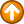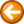CalcVar - do simple arithmetic on a variableCalcVar  \$variable,operand1,operator,operand2[[,precision][,TRIM]]

Where:

 \$variable is the name of the variable that receives the result.
 operand1 is the first operand in the equation. This operand is a decimal number that can contain a single decimal point and one or more commas before the decimal point.
 operator is the operator for the calculation. The following operators are valid:
 - Subtract operand2 from operand1.
 * Multiply operand1 by operand2.
 / Divide operand1 by operand2. The result of a division operation is always rounded down. Use the remainder operator (%) to get the remainder from a division operation.
 % Divide operand1 by operand2 and return the remainder.
 operand2 is the second operand in the equation. This operand is a decimal number that can contain a single decimal point and one or more commas before the decimal point.
 precision is an optional parameter that specifies the number of decimal places to include in the result. The default is zero.
 TRIM is and optional keyword that trims trailing zeros following the decimal point.

operand1, operator, operand2, precision and/or TRIM may be variables.

The CalcVar command performs integer or decimal arithmetic and places the result in a variable. If precision is omitted, integer arithmetic is performed.

Examples

 CalcVar \$Sum,2,+,2
 CalcVar \$Difference,8,-,4
 CalcVar \$Product,2,*,2
 CalcVar \$Quotient,16,/,4
 CalcVar \$Quotient,16.5,/,4.5,4,TRIM
 CalcVar \$Remainder,3,%,2
 CalcVar \$LineNumber,\$LineNumber,+,1
 CalcVar \$Position,\$Position,+,\$Columns

Sample Script

* CalcVar.txt

* Type 1 through 9

SetVar \$Count,1

LOOP:

type \$Count

CalcVar \$Count,\$Count,+,1

if \$Count,LT,10,LOOP:

exit